In this tutorial, we will implement transformers step-by-step and understand their implementation. There are other great tutorials on the implementation of transformers, but they usually dive into the complex parts too early, like they directly start implementing additional parts like masks and multi-head attention, but it is not very intuitional without first building the core part of the transformers.

Because of that, we will first implement a basic transformer, without any additional stuff like masking, positional encoding, multi-heads, etc. We will start with the basics and then add other stuff to it so we will understand how each part is used.

I will not go through how transformers work. If you don’t know how they are working, there is a great video for that from StatQuest.

This blog post will have a follow-up on Vision Transformers. Training transformers on language data requires big datasets and lots of computing to start seeing something useful. I am also not an NLP person, so I have very limited knowledge of that. But I know the vision. In the Vision Transformers blog, we will apply what we have learned to vision data. And to be honest, that implementation will be much simpler.

Anyway, let’s start understanding the implementation of transformers. We need to have data first. Apart from most of the other transformers implementation tutorials, we will use real data. Specifically we will useopus 100 dataset’s English to German translation. We can start our implementation by downloading the dataset. We will use HuggingFace’s datasets library for that:

import datasets
import torch
from torch import nn
import torch.nn.functional as F

torch.manual_seed(0)


Note that we have selected the first 50 data, or running the code will take a lot of time. Also, we will be using the same dataset for testing, because we are just trying to understand if our network is working. Testing on the test data will require a lot of computation which is not ideal for a tutorial.

Anyway, the loaded dataset is iterable and we can peek into it:

for data in dataset:
print(data)
break


Here you can see it returns a dictionary with de and en keys. This is perfect. But as you can imagine we need to convert the text into numbers. Let’s convert each text to a number. Like for Good Morning we want something like [2, 7]. This is easily achievable.

From now on, let’s call these words tokens. We can take all training data and put each token into a list, then we can use the indexes of each token to convert it into a number. Of course, we need to do this separately for English words and German words.

An important part here is we also need to add the <EOS> token to the target tokens. Because we will use that token to first give into the decoder later on and we will expect the network to predict <EOS> when the translation is done. If you don’t know what I am talking about, I again suggest you watch this video.

source_tokens = set()
target_tokens = set()

for data in dataset:
source = data["translation"]["en"]
target = data["translation"]["de"]

s_token_list = source.split()
t_token_list = target.split()

source_tokens.update(s_token_list)
target_tokens.update(t_token_list)

source_tokens = list(source_tokens)
target_tokens = list(target_tokens)


Let’s take a peek at the tokens:

print(source_tokens[:5])
print(target_tokens[:5])


Here you can see some English words and German words. Now we need to convert the text data to numbers using our tokens lists. For the target sequences, we will add <EOS> at the beginning and end, which we will need for the decoder part.

dataset_numeric = []
for data in dataset:
en_seq = data["translation"]["en"]
de_seq = data["translation"]["de"]

numeric_data = {}
numeric_data["en"] = [source_tokens.index(token) for token in en_seq.split()]
numeric_data["de"] = [target_tokens.index(token) for token in de_seq.split()]

numeric_data["de"].insert(0, target_tokens.index("<EOS>"))
numeric_data["de"].append(target_tokens.index("<EOS>"))

dataset_numeric.append(numeric_data)


Here we go, dataset_numeric is now a list with numeric values instead of text. You can take a look inside of it.

Now we have our data ready, we can start implementing our transformer module. The first step of transformers is that we need to have an embedding for our sequences. Let’s say our embedding dimension will be 4. So each numeric token will be mapped to 4 numbers. We can use nn.Embedding for this:

class Transformer(nn.Module):
def __init__(self, source_vocab_len, target_vocab_len, embed_dim):
super.__init__()

self.encoder_embedding = nn.Embedding(source_vocab_len, embed_dim)
self.decoder_embedding = nn.Embedding(target_vocab_len, embed_dim)


nn.Embedding is similar to a lookup table. In its first argument, you define the maximum size of this table. We will give our vocab length for that argument as that’s the maximum number of different tokens we have.

In its second argument, it asks for the embedding dimension. We will use 4 for this as we talked. Now our embeddings will take an integer and return 4 floats. For example, if we have Good Morning as our sequence and if its numeric value is [2, 7], then our encoder_embedding will convert it to something like [ [0.3, 0.4, 0.25, 0.96], [1.1, 0.15, 0.39, 0.25] ].

We can visualize how these embeddings work with this figure: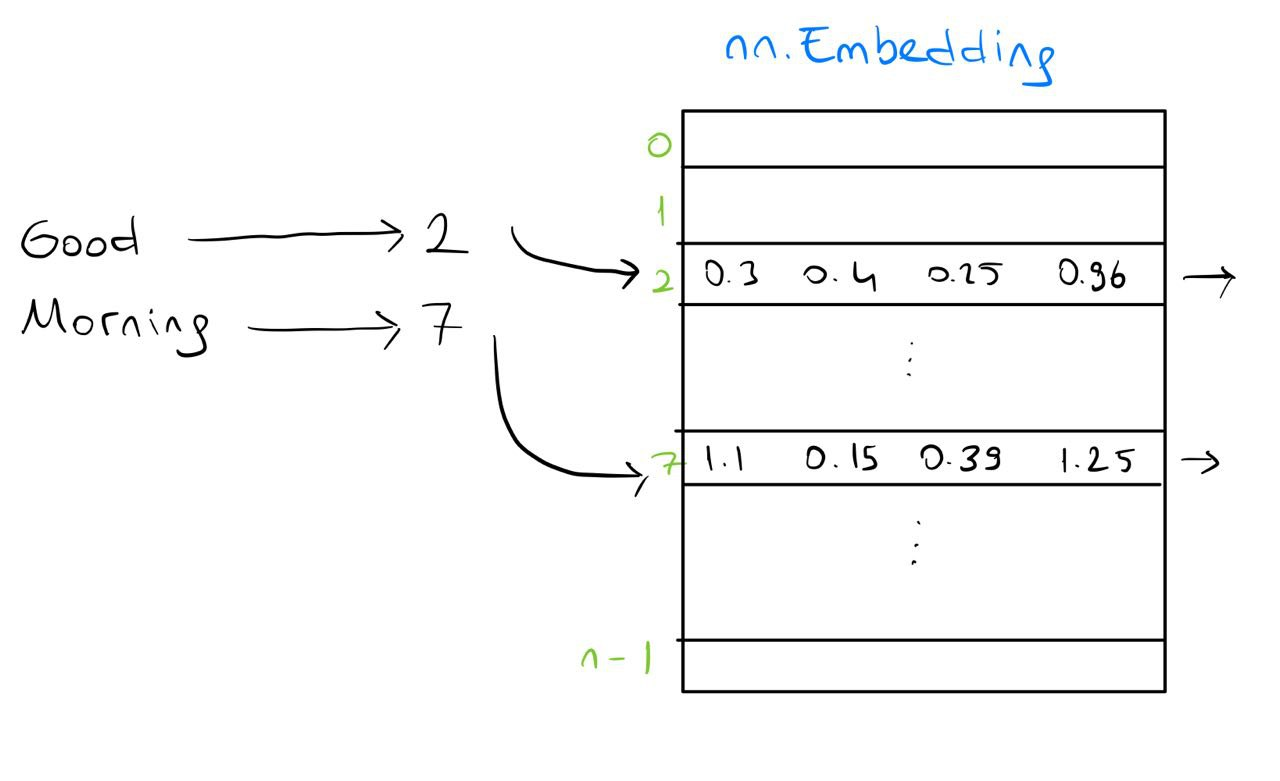The encoder will take an embedded sequence. In our case, it will take the English sequence that we want to translate. If the sequence is Good morning, we want to first find the query, key, and value for each token’s embeddings. So we will find 2 queries, 2 keys, and 2 values. One for Good, one for Morning.

An important thing to note is that the query, key, and value dimensions will be the same as the embedding dims. Since our embedding size is 4, we will those query, key, and value dimensions will be 4.

Let’s create a new module for this, called Attention:

class Attention(nn.Module):
def __init__(self, embed_dim):
super().__init__()

self.W_q = nn.Linear(embed_dim, embed_dim)
self.W_k = nn.Linear(embed_dim, embed_dim)
self.W_v = nn.Linear(embed_dim, embed_dim)

def forward(self, x, y, z):
# x, y and z will be all same for encoder attention,
# and each will be sequence of embeddings
Q = self.W_q(x)
K = self.W_k(y)
V = self.W_v(z)


This module will find the query, key, and value of each given token embedding. We will use the same Attention block for encoding all English words. This means that weights that will be multiplied by embeddings will be the same for both Good and morning.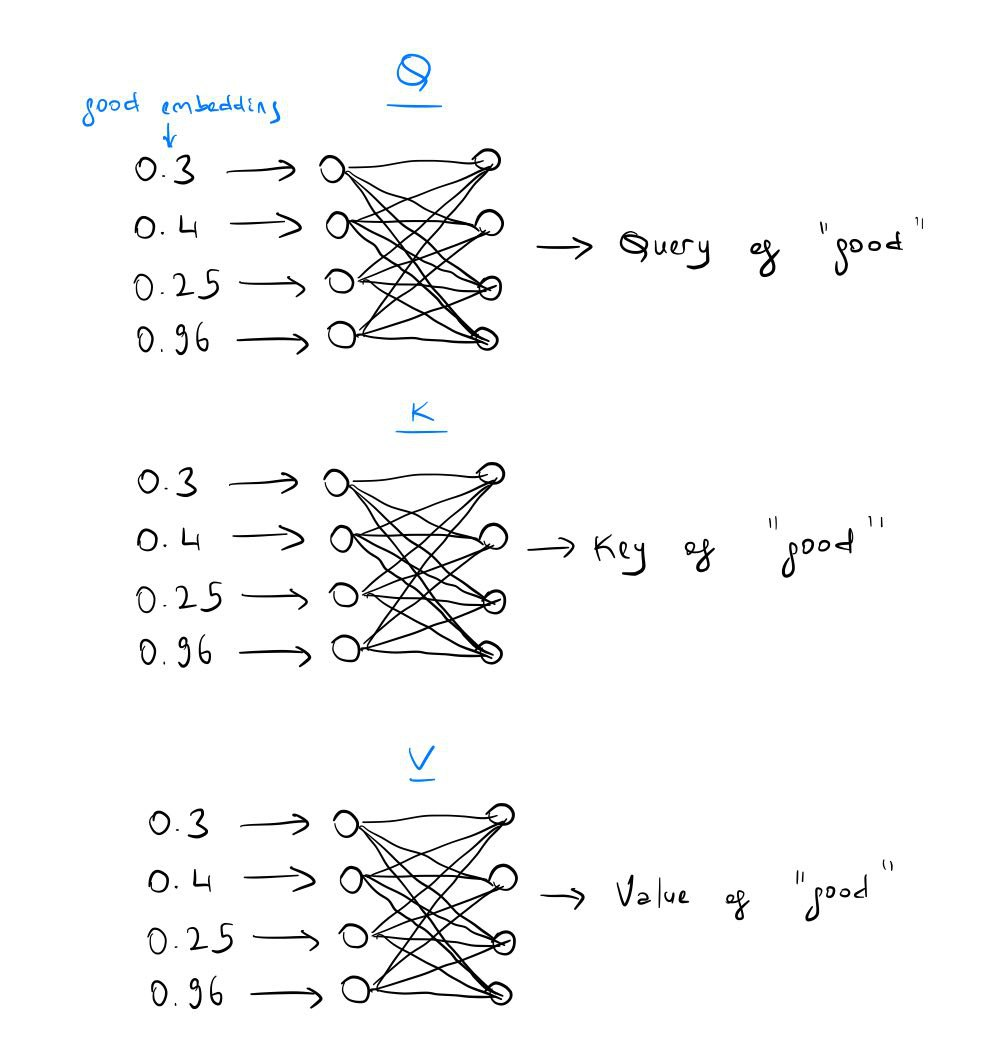Next, we will calculate the similarity between each token embedding by multiplying the query of each embedding to every other embedding’s key. This requires n^2 operations because we will calculate this for each token to every token.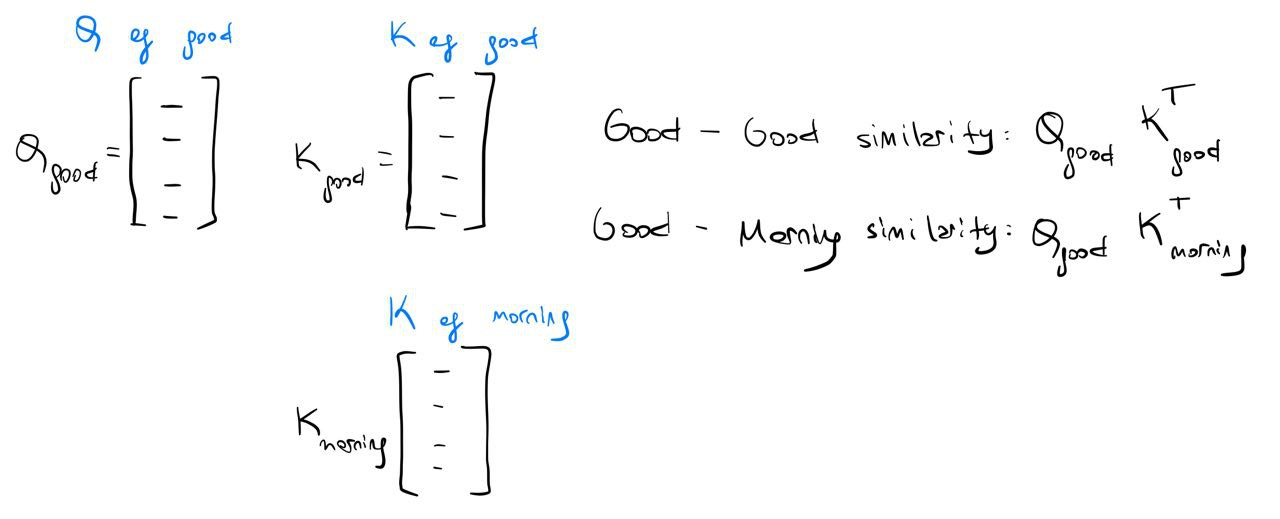We could calculate this similarity with a nested for loop, but we can achieve the same result with just Q @ K.T, and it’s faster and easier to read.

class Attention(nn.Module):
def __init__(self, embed_dim):
super().__init__()

self.W_q = nn.Linear(embed_dim, embed_dim)
self.W_k = nn.Linear(embed_dim, embed_dim)
self.W_v = nn.Linear(embed_dim, embed_dim)

def forward(self, x, y, z):
Q = self.W_q(x)
K = self.W_k(y)
V = self.W_v(z)

similarity = Q @ K.T


Now we have found the similarity, we will pass it through the softmax function to find how much similarity will affect the value of each token. We can do this by directly multiplying the softmax result with the value matrix.

class Attention(nn.Module):
def __init__(self, embed_dim):
super().__init__()

self.W_q = nn.Linear(embed_dim, embed_dim)
self.W_k = nn.Linear(embed_dim, embed_dim)
self.W_v = nn.Linear(embed_dim, embed_dim)

def forward(self, x, y, z):
Q = self.W_q(x)
K = self.W_k(y)
V = self.W_v(z)

similarity = Q @ K.T
attention = torch.softmax(similarity, dim=-1) @ V

return attention


Now our attention module is done! Although we wrote this module for the encoder part, we can also use it for the decoder part as it will be needed there too. It’s time to connect everything. Here is the architecture we are trying to build first: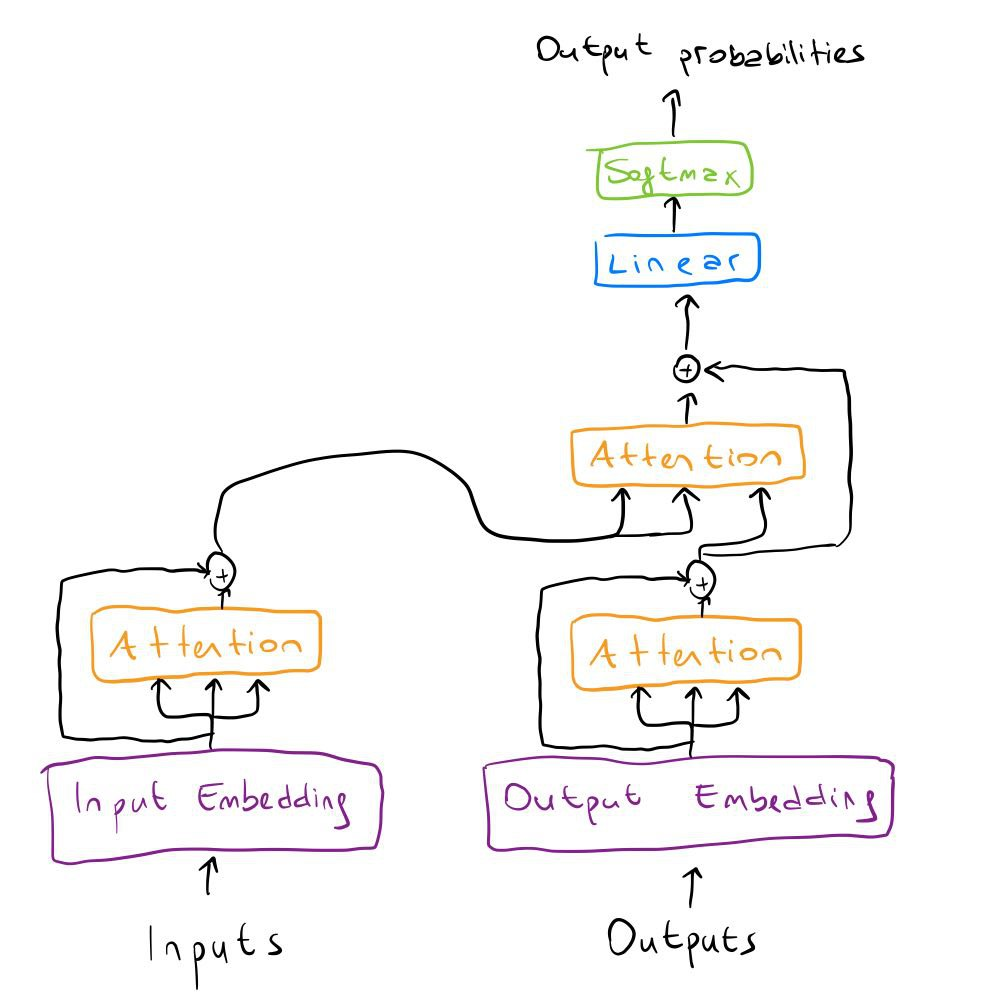class Transformer(nn.Module):
def __init__(self, source_vocab_len, target_vocab_len, embed_dim):
super().__init__()

self.encoder_embedding = nn.Embedding(source_vocab_len, embed_dim)
self.decoder_embedding = nn.Embedding(target_vocab_len, embed_dim)

self.encoder_attention = Attention(embed_dim)
self.decoder_attention = Attention(embed_dim)
self.cross_attention = Attention(embed_dim)

self.fc = nn.Linear(embed_dim, target_vocab_len)

def forward(self, source_seq, target_seq):
source_embed = self.encoder_embedding(source_seq)
target_embed = self.decoder_embedding(target_seq)

encoder_output = self.encoder_attention(source_embed, source_embed, source_embed)
decoder_output = self.decoder_attention(target_embed, target_embed, target_embed)
cross_output = self.cross_attention(decoder_output, encoder_output, encoder_output)

return self.fc(cross_output)


The fully connected layer at the end is for predicting the next token that will be outputted from the decoder part. Its output size will be target_vocab_len representing how suitable each token in the target vocabulary as the next token.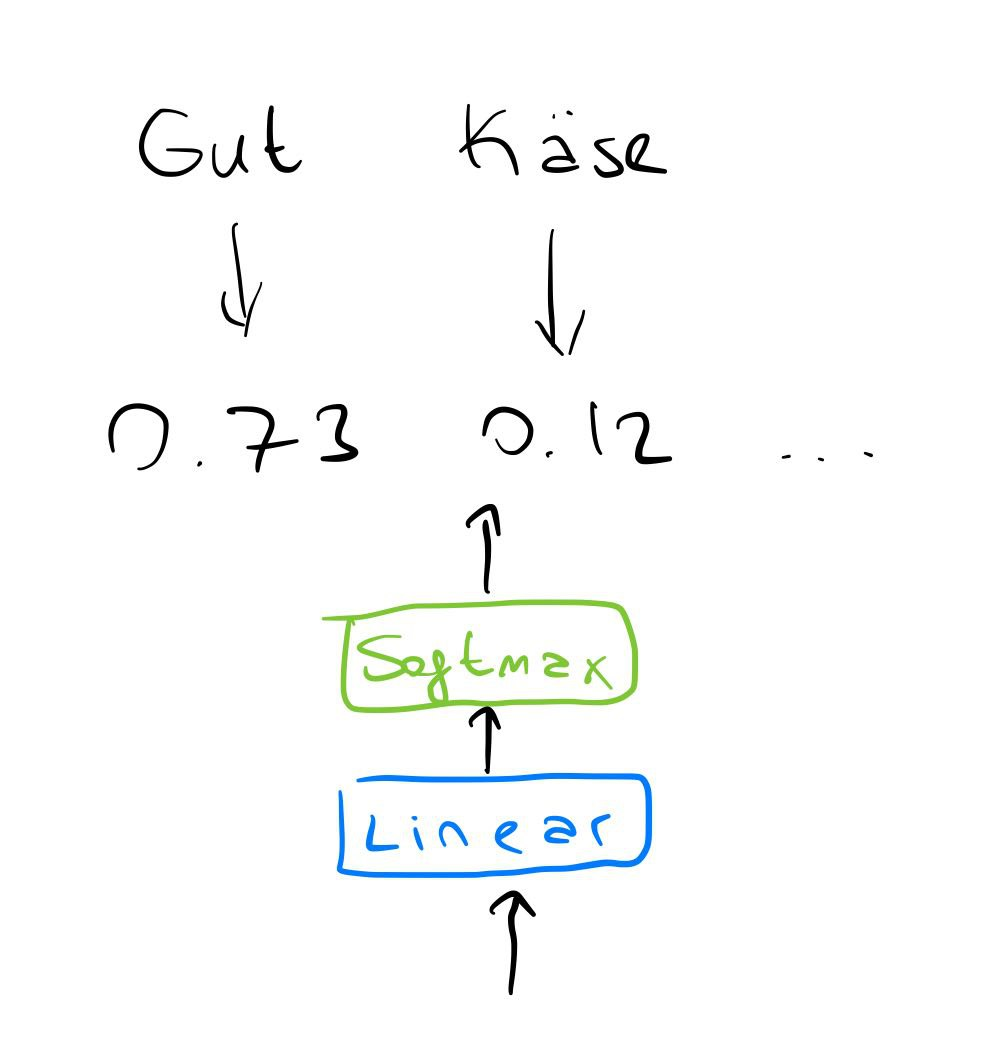Before finishing the transformer, let’s add one more thing to it: residual connections. If you look at the transformer architecture figure, you’ll see that after each attention we add residual connections. This is very easy to implement:

class Transformer(nn.Module):
def __init__(self, source_vocab_len, target_vocab_len, embed_dim):
super().__init__()

self.encoder_embedding = nn.Embedding(source_vocab_len, embed_dim)
self.decoder_embedding = nn.Embedding(target_vocab_len, embed_dim)

self.encoder_attention = Attention(embed_dim)
self.decoder_attention = Attention(embed_dim)
self.cross_attention = Attention(embed_dim)

self.fc = nn.Linear(embed_dim, target_vocab_len)

def forward(self, source_seq, target_seq):
source_embed = self.encoder_embedding(source_seq)
target_embed = self.decoder_embedding(target_seq)

encoder_output = self.encoder_attention(source_embed, source_embed, source_embed)
encoder_output += source_embed

decoder_output = self.decoder_attention(target_embed, target_embed, target_embed)
decoder_output += target_embed

cross_output = self.cross_attention(decoder_output, encoder_output, encoder_output)
cross_output += decoder_output

return self.fc(cross_output)


Now we are done with the implementation and it is time for the training loop. We will input each numeric source and target tokens up to a point in the translation, and will get a prediction of the next token. Up to a point is important, we want our network to guess the next token. So we will only give the first n tokens of the translation, and expect it to find the next token.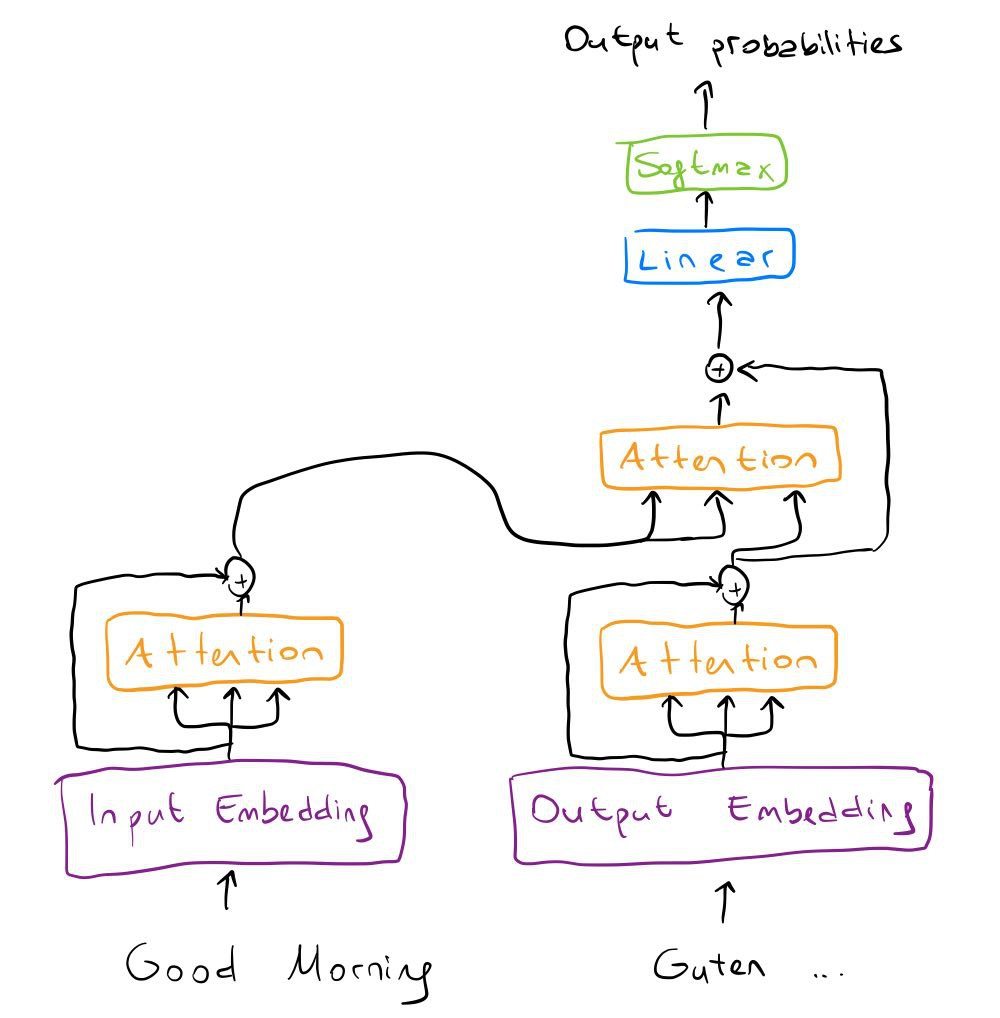We will use cross entropy as our loss function. Also, we will shift the target sequences by 1 index to the right because we are expecting the shifted targets from our network. We can think of shifted targets are our labels.

transformer = Transformer(len(source_tokens), len(target_tokens), embed_dim=4)

transformer.train()
for epoch in range(200):
losses = []
for data in dataset_numeric:
src_seq = torch.tensor(data["en"])
tgt_seq = torch.tensor(data["de"])

first_n_token = torch.randint(low=1, high=len(tgt_seq), size=(1,)).item()

output = transformer(src_seq, tgt_seq[:first_n_token])
loss = F.cross_entropy(output, tgt_seq[1 : first_n_token + 1])

loss.backward()
optimizer.step()

losses.append(loss.item())

if (epoch + 1) % 20 == 0:
print(f"Epoch: {epoch+1}, Loss: {sum(losses)}")


Now we are done! Let’s test if it works by giving the same data we trained and we expect it to give us correct the translations.

We will start with feeding <EOS> as the target sequence and will run the network again and again by adding the previous prediction to the target part. Then we will stop when we get a <EOS> token, which indicates our network thinks it finished the translation.

transformer.eval()
for data in dataset_numeric:
src_seq = torch.tensor(data["en"])

translation = torch.tensor([target_tokens.index("<EOS>")])
translation_text = []

for _ in range(40):  # we say it can be max 40 tokens per sequence
next_word_prob = transformer(src_seq, translation)[-1]
pred = torch.argmax(next_word_prob).item()
next_word = target_tokens[pred]

translation_text.append(next_word)
translation = torch.cat(
(
translation,
torch.tensor([target_tokens.index(next_word)]),
)
)

if next_word == "<EOS>":
break

en_text = " ".join([source_tokens[idx] for idx in data["en"]])
de_text = " ".join([target_tokens[idx] for idx in data["de"][1:]])
de_pred = " ".join(translation_text)

print(f"orig: {en_text}")
print(f"real: {de_text}")
print(f"pred: {de_pred}")
print("---------")


We are now done implementing the core transformer! As you can see our network has memorized our training dataset successfully.

You can see the code until this point here.

It is time to make our transformer module more powerful. Here are what are we going to do next:

1. Enable batch-processing
4. Add an option to make encoder and decoder modules multi-layer
5. Adding fully connected layers and layer norms in the encoder and decoder
6. Make the attention module multi-head

### 1. Enable batch-processing

Until now, we gave each source and target sequence one by one to the network. Of course, this is slow, and we can benefit from GPUs with batch processing.

Actually, our current implementation of the transformer module is almost capable of batch processing. All we need is to do Q @ K.transpose(1, 2) while calculating similarity scores instead of doing Q @ K.T. Because K will have the batches in its first axis.

class Attention(nn.Module):
def __init__(self, embed_dim):
super().__init__()

self.W_q = nn.Linear(embed_dim, embed_dim)
self.W_k = nn.Linear(embed_dim, embed_dim)
self.W_v = nn.Linear(embed_dim, embed_dim)

def forward(self, x, y, z):
Q = self.W_q(x)
K = self.W_k(y)
V = self.W_v(z)

similarity = Q @ K.transpose(1, 2)
attention = torch.softmax(similarity, dim=-1) @ V

return attention


Next, we need to add <PAD> tokens to our source and target sequences so that each sequence in a batch will have the same number of tokens inside. This is required because we will put different sequences into a single tensor.

The first thing we need now is to set a DataLoader that will give us batches:

dataset = datasets.load_dataset("opus100", "de-en")["train"].select(range(50))


Next, we need to modify source_tokens and target_tokens as we will be requiring <PAD> tokens inside.

source_tokens.append("<PAD>")


So far we converted all the text into numeric values before starting the training procedure. Now we will be doing this each time we load a batch instead of doing this all before starting the training. If we had lots of data that would be very expensive, better to do it batch-by-batch.

So it would be nice to have a function that takes a batch of sequences along with the tokens and returns the numeric values. Now we also want this function to add padding to our sequences to make them the same size. So we will find the sequence with the maximum number of tokens first for both source sequences and target sequences, then pad other sequences to have the same length.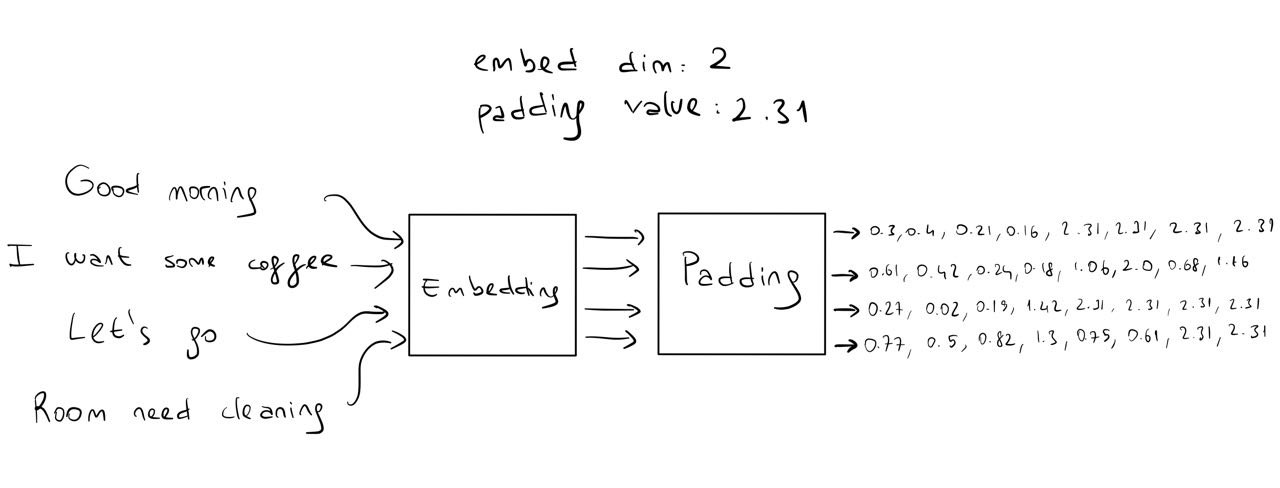def get_numeric_data(data, source_tokens, target_tokens):
data = data["translation"]

max_source_len = 0
for seq in data["en"]:
max_source_len = max(max_source_len, len(seq.split()))

max_target_len = 0
for seq in data["de"]:
max_target_len = max(max_target_len, len(seq.split()))

source_numeric_tokens = []
target_numeric_tokens = []

for s_seq, t_seq in zip(data["en"], data["de"]):
source_numeric_token = []
tokens = s_seq.split()
for token in tokens:
source_numeric_token.append(source_tokens.index(token))

torch.tensor(source_numeric_token),
)

source_numeric_tokens.append(source_numeric_token)

###

# we need to have <EOS> at the start and end for target sequences
target_numeric_token = [target_tokens.index("<EOS>")]

tokens = t_seq.split()
for token in tokens:
target_numeric_token.append(target_tokens.index(token))

target_numeric_token.append(target_tokens.index("<EOS>"))
torch.tensor(target_numeric_token),
)

target_numeric_tokens.append(target_numeric_token)



This function will return a batch of numeric sequences now, just like we want it to.

We haven’t been using the GPU yet, let’s enable it by moving our transformer to it, and then the only thing left to do is update the training loop to use DataLoader, and change the slicing axis while taking the first n token:

device = "cuda" if torch.cuda.is_available() else "cpu"

transformer = Transformer(len(source_tokens), len(target_tokens), embed_dim=4).to(device)

transformer.train()
for epoch in range(1000):
losses = []
src_seq, tgt_seq = get_numeric_data(data, source_tokens, target_tokens)
src_seq = src_seq.to(device)
tgt_seq = tgt_seq.to(device)

first_n_token = torch.randint(low=1, high=tgt_seq.shape, size=(1,)).item()

output = transformer(src_seq, tgt_seq[:, :first_n_token])
loss = F.cross_entropy(
output.view(-1, len(target_tokens)),
tgt_seq[:, 1 : first_n_token + 1].contiguous().view(-1),
)

loss.backward()
optimizer.step()

losses.append(loss.item())

if (epoch + 1) % 100 == 0:
print(f"Epoch: {epoch+1}, Loss: {sum(losses)}")


As you can see, we again managed to get a good result, but we are now much faster due to the batched inputs.

Now let’s modify the evaluation code for supporting the dataloader, then evaluate to see results.

transformer.eval()
src_seq, _ = get_numeric_data(data, source_tokens, target_tokens)
src_seq = src_seq.to(device)

translations = torch.zeros((src_seq.shape, 1), dtype=torch.int64, device=device)
translations[:] = target_tokens.index("<EOS>")

translated_texts = []
for _ in range(40):  # we say it can be max 40 tokens per sequence
next_word_probs = transformer(src_seq, translations)[:, -1, :]
preds = torch.argmax(next_word_probs, dim=-1)
next_words = [target_tokens[i] for i in preds]
translated_texts.append(next_words)

next_tokens = torch.tensor(
[target_tokens.index(w) for w in next_words],
dtype=torch.int64,
device=device,
).unsqueeze(1)

translations = torch.cat((translations, next_tokens), dim=1)

for i, text_arr in enumerate(list(zip(*translated_texts))):
if "<EOS>" in text_arr:
text_arr = text_arr[: text_arr.index("<EOS>") + 1]

en = data["translation"]["en"][i]
de = data["translation"]["de"][i]
de_pred = " ".join(text_arr)

print(f"orig: {en}")
print(f"real: {de}")
print(f"pred: {de_pred}")
print("---------")


Very good result!

You can see the code until this point here.

Now we have a problem with our batched inputs. They contain <PAD> tokens that our network might learn to attend to. We don’t want our network to attend to it. We can solve this problem by creating a mask for <PAD> tokens, and we can make the similarity scores to these tokens -inf to prevent the network from multiplying with value.

Let’s start adding a mask option to our Attention module that given a mask puts a very big negative number for similarity score:

class Attention(nn.Module):
def __init__(self, embed_dim):
super().__init__()

self.W_q = nn.Linear(embed_dim, embed_dim)
self.W_k = nn.Linear(embed_dim, embed_dim)
self.W_v = nn.Linear(embed_dim, embed_dim)

def forward(self, x, y, z, mask=None):
Q = self.W_q(x)
K = self.W_k(y)
V = self.W_v(z)

similarity = Q @ K.transpose(1, 2)

attention = torch.softmax(similarity, dim=-1) @ V
return attention


Now we need to have a mask that has True in indexes of <PAD> tokens. We can get this mask when we call the Transformer:

class Transformer(nn.Module):
def __init__(self, source_vocab_len, target_vocab_len, embed_dim):
super().__init__()

self.encoder_embedding = nn.Embedding(source_vocab_len, embed_dim)
self.decoder_embedding = nn.Embedding(target_vocab_len, embed_dim)

self.encoder_attention = Attention(embed_dim)
self.decoder_attention = Attention(embed_dim)
self.cross_attention = Attention(embed_dim)

self.fc = nn.Linear(embed_dim, target_vocab_len)

source_embed = self.encoder_embedding(source_seq)
target_embed = self.decoder_embedding(target_seq)

encoder_output = self.encoder_attention(source_embed, source_embed, source_embed, source_mask)
encoder_output += source_embed

decoder_output = self.decoder_attention(target_embed, target_embed, target_embed, target_mask)
decoder_output += target_embed

cross_output = self.cross_attention(decoder_output, encoder_output, encoder_output)
cross_output += decoder_output

return self.fc(cross_output)


Now we are all good, and we can train again and get a better result.

But we will have an additional mask. With this mask, we won’t need to take only the first n tokens of the target sequences when feeding to the network. We did it because we didn’t want our network to be able to peek ahead of the target sequence when predicting. It is trivial if it can have a look. If we give the whole target sequence at the same time, we would basically be asking for the answers while we are giving answers.

This mask will prevent the decoder from attending future tokens when we give the whole sequence to it. The method is the same, we will have a mask that has True at indexes for future tokens.

Here is the code for it and we will break it down to understand:

class Transformer(nn.Module):
def __init__(self, source_vocab_len, target_vocab_len, embed_dim):
super().__init__()

self.encoder_embedding = nn.Embedding(source_vocab_len, embed_dim)
self.decoder_embedding = nn.Embedding(target_vocab_len, embed_dim)

self.encoder_attention = Attention(embed_dim)
self.decoder_attention = Attention(embed_dim)
self.cross_attention = Attention(embed_dim)

self.fc = nn.Linear(embed_dim, target_vocab_len)

t_seq_len = target_seq.shape

source_embed = self.encoder_embedding(source_seq)
target_embed = self.decoder_embedding(target_seq)

encoder_output = self.encoder_attention(source_embed, source_embed, source_embed, source_mask)
encoder_output += source_embed

decoder_output = self.decoder_attention(target_embed, target_embed, target_embed, combined_target_mask)
decoder_output += target_embed

cross_output = self.cross_attention(decoder_output, encoder_output, encoder_output)
cross_output += decoder_output

return self.fc(cross_output)


First, we use torch.triu to create an upper triangular matrix. Our upper triangular matrix will look like thisThis matrix naturally has True in the future value for the sequence. The first token in the target sequence can only attend to itself and none other. The second token can only attend to the first token and itself, and so on.

Then, we combine target_mask and look_ahead_mask with logical or. That way we will have a single mask that both prevent attending to <PAD> tokens and future tokens for the target sequence.

We don’t need first_n_token now and we can give all the target sequences except the last token directly as it can’t peak ahead anymore:

transformer = Transformer(len(source_tokens), len(target_tokens), embed_dim=4).to(device)

transformer.train()
for epoch in range(1000):
losses = []
src_seq, tgt_seq = get_numeric_data(data, source_tokens, target_tokens)
src_seq = src_seq.to(device)
tgt_seq = tgt_seq.to(device)

loss = F.cross_entropy(
output.view(-1, len(target_tokens)),
tgt_seq[:, 1:].contiguous().view(-1),
)

loss.backward()
optimizer.step()

losses.append(loss.item())

if (epoch + 1) % 100 == 0:
print(f"Epoch: {epoch+1}, Loss: {sum(losses)}")


Now let’s test with the same evaluation code:

transformer.eval()
src_seq, _ = get_numeric_data(data, source_tokens, target_tokens)
src_seq = src_seq.to(device)

translations = torch.zeros((src_seq.shape, 1), dtype=torch.int64, device=device)
translations[:] = target_tokens.index("<EOS>")

translated_texts = []
for _ in range(40):  # we say it can be max 40 tokens per sequence
next_word_probs = transformer(
)[:, -1, :]

preds = torch.argmax(next_word_probs, dim=-1)
next_words = [target_tokens[i] for i in preds]
translated_texts.append(next_words)

next_tokens = torch.tensor(
[target_tokens.index(w) for w in next_words],
dtype=torch.int64,
device=device,
).unsqueeze(1)

translations = torch.cat((translations, next_tokens), dim=1)

for i, text_arr in enumerate(list(zip(*translated_texts))):
if "<EOS>" in text_arr:
text_arr = text_arr[: text_arr.index("<EOS>") + 1]

en = data["translation"]["en"][i]
de = data["translation"]["de"][i]
de_pred = " ".join(text_arr)

print(f"orig: {en}")
print(f"real: {de}")
print(f"pred: {de_pred}")
print("---------")


Here we are, masking is also done! It works even better, you will notice it converges to a lower loss much faster.

You can see the code until this point here.

Our Transformer model is position invariant. Meaning that it will have no difference for “Good Morning” and “Morning Good” when given. For it, both are the same sequences.

Of course, this is stupid, we somehow need to change our embeddings a little bit so that they will have some kind of a positioning embedded in them.

Let’s have a new module for positional encoding. This module will take any embedding and will return the same length embedding. I will give you the code first and then explain it.

import math

class PositionalEncoding(nn.Module):
def __init__(self, embed_dim, max_seq_len):
super().__init__()

position = torch.arange(max_seq_len).unsqueeze(1)
div_term = torch.exp(torch.arange(0, embed_dim, 2) * (-math.log(10000.0) / embed_dim))

pe = torch.zeros(max_seq_len, embed_dim)
pe[:, 0::2] = torch.sin(position * div_term)
pe[:, 1::2] = torch.cos(position * div_term)

# we use register_buffer instead of self.pe because we want
# this pe to be in the same device with transformer
self.register_buffer("pe", pe)

def forward(self, x):
# x: Tensor, of shape [batch_size, seq_len, embed_dim]
x = x + self.pe[: x.shape]
return x


Here you see the position vector first. It starts from 0 and goes to the maximum sequence length in all the sequences. The div_term is a sequence of values that are used to scale the sine and cosine functions for positional encoding. The scaling ensures that positional encoding has a more meaningful gradient across dimensions.

Then we start our positional encoding as pe. Even indices of pe (0, 2, 4,…) get the sine of the product of position and div_term, while the odd indices (1, 3, 5,…) get the cosine.

I know this part is a little bit complex but in reality, it just adds a unique pattern to each position in a sequence. The reason sine and cosine functions are used is because they ensure that, even for large positions, the positional encodings don’t blow up in magnitude. Also, everything should be much clearer if you already watched the video I suggested to you at the beginning of this post.

Next, we want to use this module in our transformer module to encode our embeddings. But as you can see this expects maximum sequence length, so we need to find it first. The best way to find it is when we are finding all source and target tokens. In fact, let’s make it a function that returns the maximum sequence length as well:

def tokenizer(dataset):
source_tokens = set()
target_tokens = set()

max_seq_len = 0
for data in dataset:
s = data["translation"]["en"]
t = data["translation"]["de"]

s_token_list = s.split()
t_token_list = t.split()
max_seq_len = max(max_seq_len, len(s_token_list), len(t_token_list))

source_tokens.update(s_token_list)
target_tokens.update(t_token_list)

source_tokens = list(source_tokens)
target_tokens = list(target_tokens)

# +2 for two <EOS> in target sequences
max_seq_len += 2

return source_tokens, target_tokens, max_seq_len


Now the new transformer module:

class Transformer(nn.Module):
def __init__(self, source_vocab_len, target_vocab_len, embed_dim, max_seq_len):
super().__init__()

self.encoder_embedding = nn.Embedding(source_vocab_len, embed_dim)
self.decoder_embedding = nn.Embedding(target_vocab_len, embed_dim)
self.positional_encoding = PositionalEncoding(embed_dim, max_seq_len)

self.encoder_attention = Attention(embed_dim)
self.decoder_attention = Attention(embed_dim)
self.cross_attention = Attention(embed_dim)

self.fc = nn.Linear(embed_dim, target_vocab_len)

t_seq_len = target_seq.shape

source_embed = self.positional_encoding(self.encoder_embedding(source_seq))
target_embed = self.positional_encoding(self.decoder_embedding(target_seq))

encoder_output = self.encoder_attention(source_embed, source_embed, source_embed, source_mask)
encoder_output += source_embed

decoder_output = self.decoder_attention(target_embed, target_embed, target_embed, combined_target_mask)
decoder_output += target_embed

cross_output = self.cross_attention(decoder_output, encoder_output, encoder_output)
cross_output += decoder_output

return self.fc(cross_output)


Let’s put everything together and train again:

source_tokens, target_tokens, max_seq_len = tokenizer(dataset)

transformer = Transformer(len(source_tokens), len(target_tokens), embed_dim=4, max_seq_len=max_seq_len).to(device)

transformer.train()
for epoch in range(1000):
losses = []
src_seq, tgt_seq = get_numeric_data(data, source_tokens, target_tokens)
src_seq = src_seq.to(device)
tgt_seq = tgt_seq.to(device)

loss = F.cross_entropy(
output.view(-1, len(target_tokens)),
tgt_seq[:, 1:].contiguous().view(-1),
)

loss.backward()
optimizer.step()

losses.append(loss.item())

if (epoch + 1) % 100 == 0:
print(f"Epoch: {epoch+1}, Loss: {sum(losses)}")


Now we have finished the positional encoding.

You can see the code until this point here.

### 4. Add option to make encoder and decoder modules multi-layer

Here comes the easiest part. Adding more layers is simply connecting the output of the encoder to the input of the next encoder and the same thing for the decoder. It is just to make our network more powerful by having more parameters.

If our number of layers is 2, the architecture will look like this: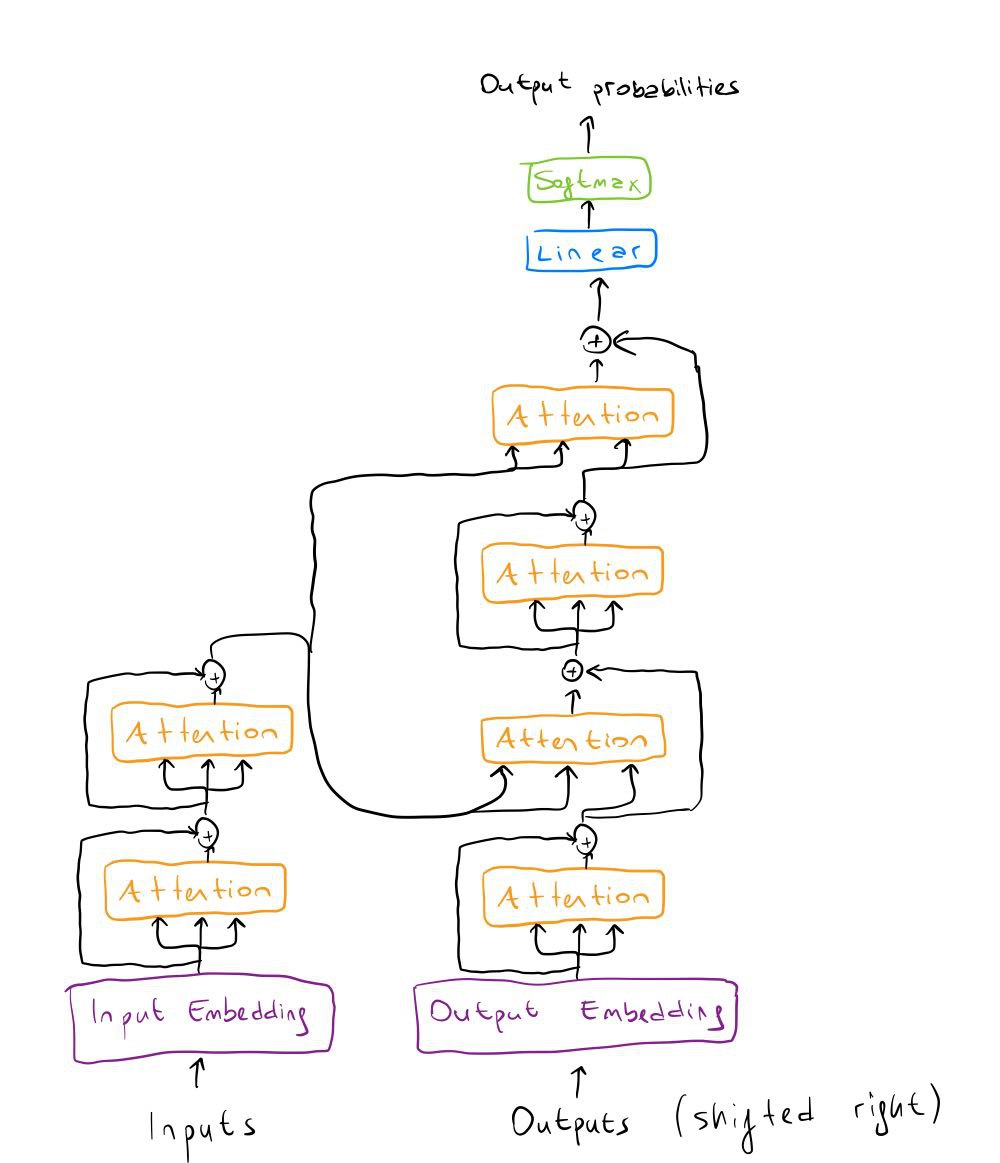It is very easy! Let’s start by making the encoder and decoder parts separate modules so it will be easier to stack them up.

class Encoder(nn.Module):
def __init__(self, embed_dim):
super().__init__()

self.attn = Attention(embed_dim)

out = self.attn(x, x, x, mask)
x = out + x

return x

class Decoder(nn.Module):
def __init__(self, embed_dim):
super().__init__()

self.attn = Attention(embed_dim)
self.cross_attn = Attention(embed_dim)

out = self.attn(x, x, x, mask)
x = out + x

out = self.cross_attn(x, y, y, mask=None)
x = out + x

return x


Now we have Encoder and Decoder as different modules and stacking up will be very easy. We will just write a loop to give the output of one layer to another layer.

class Transformer(nn.Module):
def __init__(
self,
source_vocab_len,
target_vocab_len,
embed_dim,
num_encoder_layers=6,
num_decoder_layers=6,
max_seq_len=1024,
):
super().__init__()

self.encoder_embedding = nn.Embedding(source_vocab_len, embed_dim)
self.decoder_embedding = nn.Embedding(target_vocab_len, embed_dim)
self.positional_encoding = PositionalEncoding(embed_dim, max_seq_len)

self.encoders = nn.ModuleList([Encoder(embed_dim) for _ in range(num_encoder_layers)])
self.decoders = nn.ModuleList([Decoder(embed_dim) for _ in range(num_decoder_layers)])

self.fc = nn.Linear(embed_dim, target_vocab_len)

t_seq_len = target_seq.shape

source_embed = self.positional_encoding(self.encoder_embedding(source_seq))
target_embed = self.positional_encoding(self.decoder_embedding(target_seq))

encoder_out = source_embed
for encoder in self.encoders:

decoder_out = target_embed
for decoder in self.decoders:

return self.fc(decoder_out)


Here it is! Now we completed the multi-layer part of the transformers. Let’s train again to see the effect of the layers. We can expect it to be a little bit slower but much more capable.

transformer = Transformer(
len(source_tokens),
len(target_tokens),
embed_dim=4,
num_encoder_layers=3,
num_decoder_layers=3,
max_seq_len=max_seq_len,
).to(device)

transformer.train()
for epoch in range(1000):
losses = []
src_seq, tgt_seq = get_numeric_data(data, source_tokens, target_tokens)
src_seq = src_seq.to(device)
tgt_seq = tgt_seq.to(device)

loss = F.cross_entropy(
output.view(-1, len(target_tokens)),
tgt_seq[:, 1:].contiguous().view(-1),
)

loss.backward()
optimizer.step()

losses.append(loss.item())

if (epoch + 1) % 100 == 0:
print(f"Epoch: {epoch+1}, Loss: {sum(losses)}")


Now let’s test it:

transformer.eval()
src_seq, _ = get_numeric_data(data, source_tokens, target_tokens)
src_seq = src_seq.to(device)

translations = torch.zeros((src_seq.shape, 1), dtype=torch.int64, device=device)
translations[:] = target_tokens.index("<EOS>")

translated_texts = []
for _ in range(40):  # we say it can be max 40 tokens per sequence
next_word_probs = transformer(
)[:, -1, :]

preds = torch.argmax(next_word_probs, dim=-1)
next_words = [target_tokens[i] for i in preds]
translated_texts.append(next_words)

next_tokens = torch.tensor(
[target_tokens.index(w) for w in next_words],
dtype=torch.int64,
device=device,
).unsqueeze(1)

translations = torch.cat((translations, next_tokens), dim=1)

for i, text_arr in enumerate(list(zip(*translated_texts))):
if "<EOS>" in text_arr:
text_arr = text_arr[: text_arr.index("<EOS>") + 1]

en = data["translation"]["en"][i]
de = data["translation"]["de"][i]
de_pred = " ".join(text_arr)

print(f"orig: {en}")
print(f"real: {de}")
print(f"pred: {de_pred}")
print("---------")


Works very well again.

You can see the code until this point here.

### 5. Adding fully connected layers and layer norms in encoder and decoders

We are going to add some fully connected layers in our encoder, decoder, and module to make the network a little bit more powerful. Also, we will add layer normalizations to stabilize the training process.

class Attention(nn.Module):
def __init__(self, embed_dim):
super().__init__()

self.W_q = nn.Linear(embed_dim, embed_dim)
self.W_k = nn.Linear(embed_dim, embed_dim)
self.W_v = nn.Linear(embed_dim, embed_dim)

self.linear_out = nn.Linear(embed_dim, embed_dim)

def forward(self, x, y, z, mask=None):
Q = self.W_q(x)
K = self.W_k(y)
V = self.W_v(z)

similarity = Q @ K.transpose(1, 2)

attention = torch.softmax(similarity, dim=-1) @ V
output = self.linear_out(attention)

return output

class Encoder(nn.Module):
def __init__(self, embed_dim, ff_dim):
super().__init__()

self.attn = Attention(embed_dim)
self.norm1 = nn.LayerNorm(embed_dim)

self.ff1 = nn.Linear(embed_dim, ff_dim)
self.ff2 = nn.Linear(ff_dim, embed_dim)
self.norm2 = nn.LayerNorm(embed_dim)

out = self.attn(x, x, x, mask)
x = out + x
x = self.norm1(x)

out = F.relu(self.ff1(x))
out = self.ff2(out)

x = out + x
x = self.norm2(x)
return x

class Decoder(nn.Module):
def __init__(self, embed_dim, ff_dim):
super().__init__()

self.attn = Attention(embed_dim)
self.norm1 = nn.LayerNorm(embed_dim)

self.cross_attn = Attention(embed_dim)
self.norm2 = nn.LayerNorm(embed_dim)

self.ff1 = nn.Linear(embed_dim, ff_dim)
self.ff2 = nn.Linear(ff_dim, embed_dim)
self.norm3 = nn.LayerNorm(embed_dim)

out = self.attn(x, x, x, mask)
x = out + x
x = self.norm1(x)

out = self.cross_attn(x, y, y, mask=None)
x = out + x
x = self.norm2(x)

out = F.relu(self.ff1(x))
out = self.ff2(out)

x = out + x
x = self.norm3(x)
return x

class Transformer(nn.Module):
def __init__(
self,
source_vocab_len,
target_vocab_len,
embed_dim,
num_encoder_layers=6,
num_decoder_layers=6,
ff_dim=2048,
max_seq_len=1024,
):
super().__init__()

self.encoder_embedding = nn.Embedding(source_vocab_len, embed_dim)
self.decoder_embedding = nn.Embedding(target_vocab_len, embed_dim)
self.positional_encoding = PositionalEncoding(embed_dim, max_seq_len)

self.encoders = nn.ModuleList([Encoder(embed_dim, ff_dim=ff_dim) for _ in range(num_encoder_layers)])
self.decoders = nn.ModuleList([Decoder(embed_dim, ff_dim=ff_dim) for _ in range(num_decoder_layers)])

self.fc = nn.Linear(embed_dim, target_vocab_len)

t_seq_len = target_seq.shape

source_embed = self.positional_encoding(self.encoder_embedding(source_seq))
target_embed = self.positional_encoding(self.decoder_embedding(target_seq))

encoder_out = source_embed
for encoder in self.encoders:

decoder_out = target_embed
for decoder in self.decoders:

return self.fc(decoder_out)


Now let’s train and test it:

transformer = Transformer(
len(source_tokens),
len(target_tokens),
embed_dim=4,
num_encoder_layers=3,
num_decoder_layers=3,
ff_dim=128,
max_seq_len=max_seq_len,
).to(device)

transformer.train()
for epoch in range(2000):
losses = []
src_seq, tgt_seq = get_numeric_data(data, source_tokens, target_tokens)
src_seq = src_seq.to(device)
tgt_seq = tgt_seq.to(device)

loss = F.cross_entropy(
output.view(-1, len(target_tokens)),
tgt_seq[:, 1:].contiguous().view(-1),
)

loss.backward()
optimizer.step()

losses.append(loss.item())

if (epoch + 1) % 100 == 0:
print(f"Epoch: {epoch+1}, Loss: {sum(losses)}")

transformer.eval()
src_seq, _ = get_numeric_data(data, source_tokens, target_tokens)
src_seq = src_seq.to(device)

translations = torch.zeros((src_seq.shape, 1), dtype=torch.int64, device=device)
translations[:] = target_tokens.index("<EOS>")

translated_texts = []
for _ in range(40):  # we say it can be max 40 tokens per sequence
next_word_probs = transformer(
)[:, -1, :]

preds = torch.argmax(next_word_probs, dim=-1)
next_words = [target_tokens[i] for i in preds]
translated_texts.append(next_words)

next_tokens = torch.tensor(
[target_tokens.index(w) for w in next_words],
dtype=torch.int64,
device=device,
).unsqueeze(1)

translations = torch.cat((translations, next_tokens), dim=1)

for i, text_arr in enumerate(list(zip(*translated_texts))):
if "<EOS>" in text_arr:
text_arr = text_arr[: text_arr.index("<EOS>") + 1]

en = data["translation"]["en"][i]
de = data["translation"]["de"][i]
de_pred = " ".join(text_arr)

print(f"orig: {en}")
print(f"real: {de}")
print(f"pred: {de_pred}")
print("---------")



As it can be seen, it still works great. We have increased the number of epochs a little bit because we now have many more parameters to train.

You can see the code until this point here.

Now it’s time for the last change: Multi-head attention.

### 6. Make attention module multi-head

By making the attention module multi-head, we actually mean we will separate our input embeddings into num_heads different matrices, process them in the attention layer individually, and merge them again at the end.

For example, if we have a sequence with 8 tokens, our embedding dimension is 6 and we want the number of heads to be 2, we will first have an 8x6 matrix fed into the attention module and after finding the query, key, and value for this sequence, we will divide this matrix to have the size 2x8x3, where 2 is the number of heads, 8 is the sequence length and 3 is the output of embed_dim / num_heads. Then we will calculate the similarity score for each of the heads and at the end, we will concat those to have the size 8x6.

By processing the heads individually, our network has more capabilities, so we expect better results.

You can understand the operations with this figure better: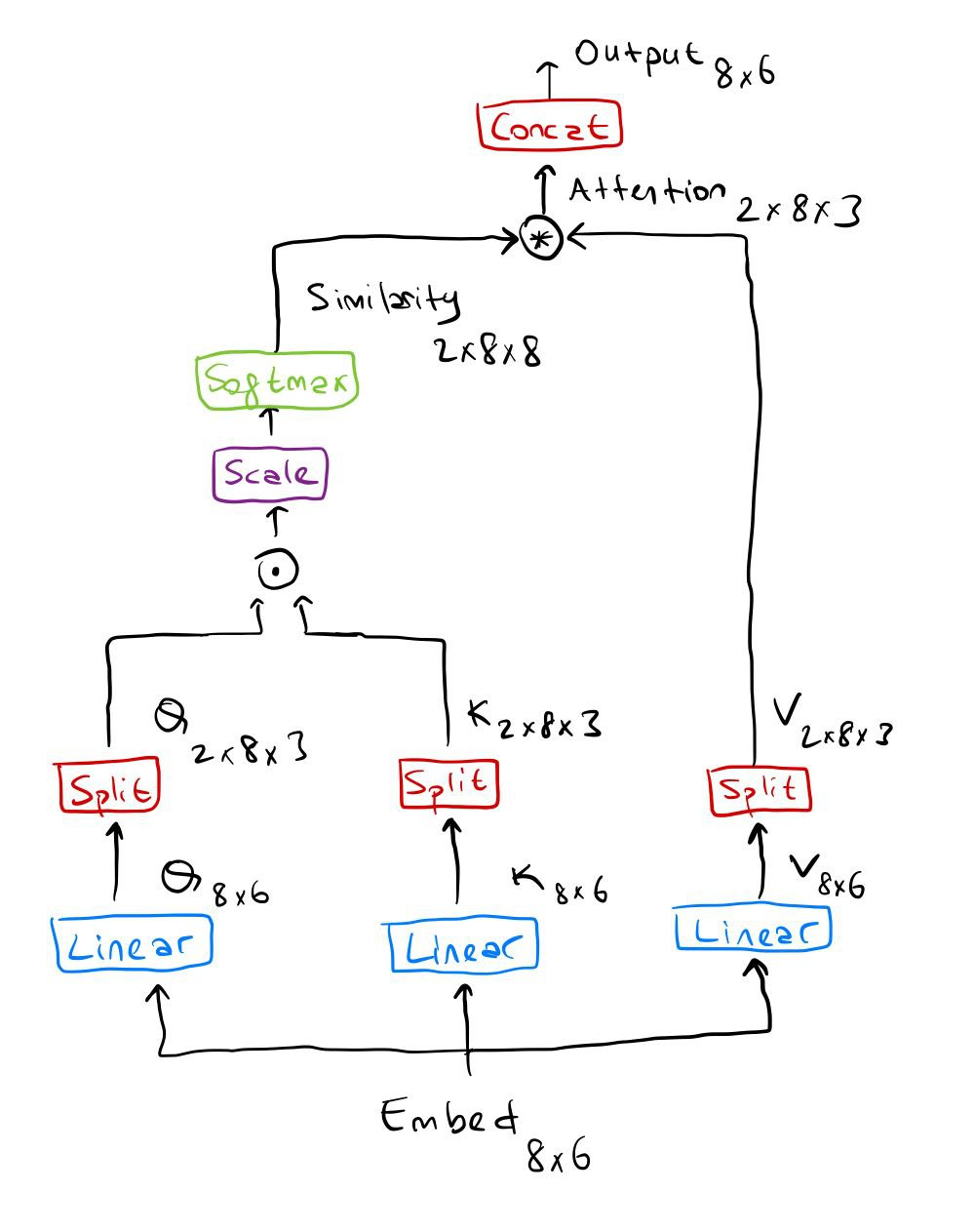And before writing the code, let’s make an additional very small change by dividing our similarity score by a value. This value is the square root of embed_dim / num_heads and the only reason we do this is just to make everything a bit more numerically stable and avoid very big numbers being outputted. This operation is called the scale and you can see it in the scaled dot-product attention figures on the web.

Without further ado, here is the implementation of this final change. First, we need to modify the attention module, and let’s rename it to MultiHeadAttention:

class MultiHeadAttention(nn.Module):
super().__init__()

assert embed_dim % num_heads == 0, "embed_dim must be divisible by num_heads to be able to seperate the matrix"

self.W_q = nn.Linear(embed_dim, embed_dim)
self.W_k = nn.Linear(embed_dim, embed_dim)
self.W_v = nn.Linear(embed_dim, embed_dim)

self.linear_out = nn.Linear(embed_dim, embed_dim)

def forward(self, x, y, z, mask=None):
N, seq_len, embed_size = x.shape

Q = self.W_q(x)
K = self.W_k(y)
V = self.W_v(z)

similarity = (Q @ K.transpose(2, 3)) / (self.head_dim**0.5)

attention = torch.softmax(similarity, dim=-1) @ V
attention = attention.transpose(1, 2).contiguous().view(N, seq_len, embed_size)
output = self.linear_out(attention)

return output


Next, the encoder and decoder layers will take the num_heads argument and pass it to MultiHeadAttention:

class Encoder(nn.Module):
super().__init__()

self.norm1 = nn.LayerNorm(embed_dim)

self.ff1 = nn.Linear(embed_dim, ff_dim)
self.ff2 = nn.Linear(ff_dim, embed_dim)
self.norm2 = nn.LayerNorm(embed_dim)

out = self.attn(x, x, x, mask)
x = out + x
x = self.norm1(x)

out = F.relu(self.ff1(x))
out = self.ff2(out)

x = out + x
x = self.norm2(x)
return x

class Decoder(nn.Module):
super().__init__()

self.norm1 = nn.LayerNorm(embed_dim)

self.norm2 = nn.LayerNorm(embed_dim)

self.ff1 = nn.Linear(embed_dim, ff_dim)
self.ff2 = nn.Linear(ff_dim, embed_dim)
self.norm3 = nn.LayerNorm(embed_dim)

out = self.attn(x, x, x, mask)
x = out + x
x = self.norm1(x)

out = self.cross_attn(x, y, y, mask=None)
x = out + x
x = self.norm2(x)

out = F.relu(self.ff1(x))
out = self.ff2(out)

x = out + x
x = self.norm3(x)
return x


Next, we will update the Transformer module. We also have to modify our masks to reflect the changes:

class Transformer(nn.Module):
def __init__(
self,
source_vocab_len,
target_vocab_len,
embed_dim,
num_encoder_layers=6,
num_decoder_layers=6,
ff_dim=2048,
max_seq_len=1024,
):
super().__init__()

self.encoder_embedding = nn.Embedding(source_vocab_len, embed_dim)
self.decoder_embedding = nn.Embedding(target_vocab_len, embed_dim)
self.positional_encoding = PositionalEncoding(embed_dim, max_seq_len)

self.encoders = nn.ModuleList(
)
self.decoders = nn.ModuleList(
)

self.fc = nn.Linear(embed_dim, target_vocab_len)

# we unsqueeze it one more time since matrix will be bigger with num_heads,
# then transpose to put the 1 in the correct place

t_seq_len = target_seq.shape
# reason we expand it is to make the NxSxS array to Nx(num_head)xSxS or resulting process is wrong

source_embed = self.positional_encoding(self.encoder_embedding(source_seq))
target_embed = self.positional_encoding(self.decoder_embedding(target_seq))

encoder_out = source_embed
for encoder in self.encoders:

decoder_out = target_embed
for decoder in self.decoders:

return self.fc(decoder_out)


And now let’s train it:

transformer = Transformer(
len(source_tokens),
len(target_tokens),
embed_dim=4,
num_encoder_layers=3,
num_decoder_layers=3,
ff_dim=128,
max_seq_len=max_seq_len,
).to(device)

transformer.train()
for epoch in range(2000):
losses = []
src_seq, tgt_seq = get_numeric_data(data, source_tokens, target_tokens)
src_seq = src_seq.to(device)
tgt_seq = tgt_seq.to(device)

loss = F.cross_entropy(
output.view(-1, len(target_tokens)),
tgt_seq[:, 1:].contiguous().view(-1),
)

loss.backward()
optimizer.step()

losses.append(loss.item())

if (epoch + 1) % 100 == 0:
print(f"Epoch: {epoch+1}, Loss: {sum(losses)}")

transformer.eval()
src_seq, _ = get_numeric_data(data, source_tokens, target_tokens)
src_seq = src_seq.to(device)

translations = torch.zeros((src_seq.shape, 1), dtype=torch.int64, device=device)
translations[:] = target_tokens.index("<EOS>")

translated_texts = []
for _ in range(40):  # we say it can be max 40 tokens per sequence
next_word_probs = transformer(
)[:, -1, :]

preds = torch.argmax(next_word_probs, dim=-1)
next_words = [target_tokens[i] for i in preds]
translated_texts.append(next_words)

next_tokens = torch.tensor(
[target_tokens.index(w) for w in next_words],
dtype=torch.int64,
device=device,
).unsqueeze(1)

translations = torch.cat((translations, next_tokens), dim=1)

for i, text_arr in enumerate(list(zip(*translated_texts))):
if "<EOS>" in text_arr:
text_arr = text_arr[: text_arr.index("<EOS>") + 1]

en = data["translation"]["en"][i]
de = data["translation"]["de"][i]
de_pred = " ".join(text_arr)

print(f"orig: {en}")
print(f"real: {de}")
print(f"pred: {de_pred}")
print("---------")


And this was our last modification to the code. Now you know the details of implementing the transformer architecture. We have fully implemented transformer architecture step-by-step.

You can see the fully functional transformer code here.

In the next post, we will learn about ViT, and then implement it. It will be much simpler because we will only require the encoder part and the positional encoding part will be much more easy to implement. I will put the link here when it’s posted.